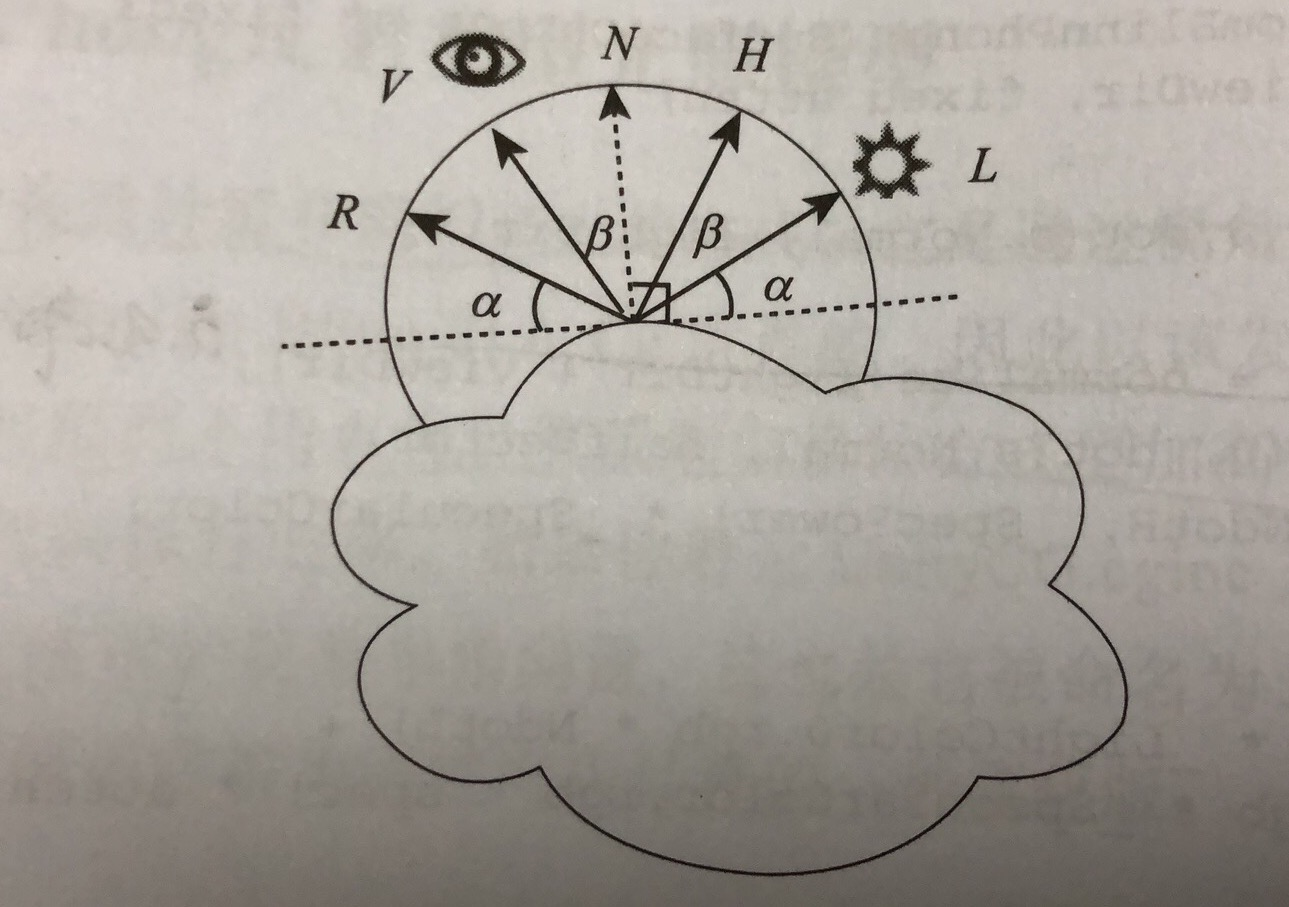Unity 表面着色器为开发者提供了一层简单快速的编写 Shader 的方式，对开发者来说隐藏了光照模型这些复杂的的概念，但是有时候 Unity 自带的光照模型往往不能满足我们的需求，而需要自己定义光照模型。所以接下来就一起看看 Unity 中常见的光照模型函数。

Lambert 光照模型

• $Iad = kd \times Ia$

• Ia 是环境光的强度

• kd 为材质对环境光的反射系数(0 < kd < 1)

• $Iad = kd \times Il \times cosθ$

• Il 是方向光的强度

• kd 为材质对环境光的反射系数(0 < kd < 1)

• θ 是入射光方向和顶点法线的夹角。当夹角为 0°，说明入射光平行于法线(垂直于表面)，此时反射强度最大；当夹角为 90° 时，说明入射光同表面顶点切线平行，此时物体不会反射任何光线。

cosθ 等价于顶点单位法向量 N 与从顶点指向光源的单位向量 L 的点积，所以有 $Iad = kd \times Il \times (N \cdot L)$

$Idiff = kd \times Ia + kd \times Il \times (N·L)$

Half Lambert 光照模型

Half Lambert 用来给在比较暗的区域显示物体。实现也很简单，代码如下：

Phong 光照模型

Phong 光照模型表面某点处的镜面反射光强：

• $Is = ks \times Il \times (R \cdot V) ^ p$

• ks 是材质的镜面反射系数

• Il 是光强

• R 为反射光的方向

• V 表示从顶点到视点的方向

• p 是高光指数，p 越大反射越集中，当慢慢视线方向偏离反射方向光线开始慢慢衰减，反之 p 越小观察到的光斑区域也就越小，反射光强度也很弱。

$R + L = 2 \times N \times (N \cdot L)$ => $R = 2 \times N \times (N \cdot L) - L$

$Is = ks \times Il \times ((2 \times N \times (N \cdot L) - L) \cdot V) ^ p$

inline fixed4 PhongLight (SurfaceOutput s, half3 viewDir, UnityLight light)

Phong 光照模型时假设反射型表面的最终光照强度取决于两个因素：漫反射颜色和光线的反射值，所以有：

• $I = D + S$

• D 是根据 Lambert 计算的漫反射部分

• S 就是上面我们介绍的镜面反射部分

BlinnPhong 光照模型

Blinn 是一种高效计算和模拟高光的方式，它是通过视角方向和光线方向构成的半角向量完成的。BlinnPhong 光照模型则是混合和了 Lambert 的漫反射和 Blinn 计算高光的模式，渲染有时比 Phong 高光更柔和、更平滑，此外它的处理速度相当快。

• $Ibp = ks \times Il \times (N \cdot H) ^ p$

• ks 是材质的镜面反射系数

• Il 是光强

• N 为入射点的单位法向量

• H 表示光线方向和视角方向的半角向量

• p 是高光指数，p 越大反射越集中，当慢慢视线方向偏离反射方向光线开始慢慢衰减，反之 p 越小观察到的光斑区域也就越小，反射光强度也很弱。

H = normalize(V, L)